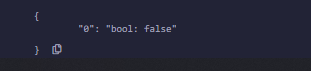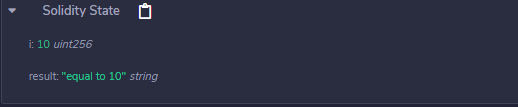Related Articles
Solidity – Decision Making Statements
• Last Updated : 10 Jul, 2020

Decision making in programming is used when we have to adopt one out of a given set of paths for program flow. For this purpose, conditional statements are used which allows the program to execute the piece of code when the condition fulfills. Solidity uses control statements to control the flow of execution of the program to advance and branch-based changes to the state. Following conditional statements are provided by Solidity.

### If statement

This is the most basic conditional statement. It is used to make a decision whether the statement or block of code will be executed or not. If the condition is true the statements will be executed, else no statement will execute.

Syntax:

```if (condition) {
statement or block of code to be executed if the condition is True
}
```

Example: In the below example, the contract Types declares an unsigned integer state variable and a function to demonstrate the execution of if statement.

## Solidity

 `// Solidity program to ``// demonstrate the ``// use of 'if statement'``pragma solidity ^0.5.0; ``  ` `// Creating a contract``contract` `Types { `` ` `    ``// Declaring state variable``    ``uint i = 10;`` ` `    ``// Defining function to ``    ``// demonstrate use of ``    ``// 'if statement'``    ``function decision_making(``    ``) ``public` `returns(``bool``){``        ``if``(i<10){``            ``return` `true``;``        ``}``    ``}``     ` `}`

Output :### if…else statement

This statement is the next form of conditional statement which allows the program to execute in a more controlled way. Here if the condition is true then the, if block is executed while if the condition is false then else block, is executed.

Syntax:

```if (condition) {
statement or block of code to be executed if condition is True
} else {
statement or block of code to be executed if condition is False
}
```

Example: In the below example, the contract Types declares state variable and a function to demonstrate the execution of the if-else statement.

## Solidity

 `// Solidity program to ``// demonstrate the use of``// 'if...else' statement``pragma solidity ^0.5.0; ``  ` `// Creating a contract``contract` `Types { `` ` `    ``// Declaring state variables``    ``uint i = 10;``    ``bool` `even;`` ` `    ``// Defining function to ``    ``// demonstrate the use of``    ``// 'if...else statement'``    ``function decision_making(``    ``) ``public` `payable returns(``bool``){``        ``if``(i%2 == 0){``            ``even = ``true``;``        ``}``        ``else``{``            ``even = ``false``;``        ``}``        ``return` `even;``    ``}``     ` `}`

Output :### if…else if…else statement

This is a modified form of if…else conditional statement which is used to make a decision among several options. The statements start execution from if statement and as the condition of any if block is true the block of code associated with it is executed and rest if are skipped, and if none of the condition is true then else block executes.

Syntax:

```if (condition) {
statement or block of code to be executed if the condition is True
} else if (condition 2) {
statement or block of code to be executed if the condition of else...if is True
} else {
statement or block of code to be executed if none of the condition is True
}
```

Example: In the below example, the contract Types declares an unsigned integer state variable and a function to demonstrate the execution of the if-else if-else statement.

## Solidity

 `// Solidity program to ``// demonstrate the use``// of 'if-else if-else statement'``pragma solidity ^0.5.0; `` ` `// Creating a contract ``contract` `Types { `` ` `    ``// Declaring state variables``    ``uint i = 10;``    ``string result;`` ` `    ``// Defining function to ``    ``// demonstrate the use``    ``// of 'if...else if...else ``    ``// statement'``    ``function decision_making(``    ``) ``public` `returns(string memory){``        ``if``(i<10){``            ``result = ``"less than 10"``;``        ``}``        ``else` `if``(i == 10){``            ``result = ``"equal to 10"``;``        ``}``        ``else``{``            ``result = ``"greater than 10"``;``        ``}``        ``return` `result;``    ``}``     ` `}`

Output :My Personal Notes arrow_drop_up